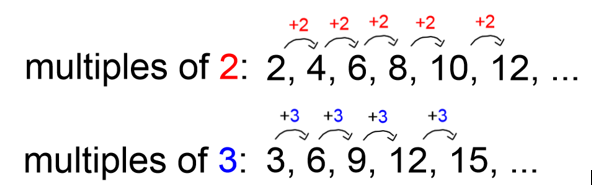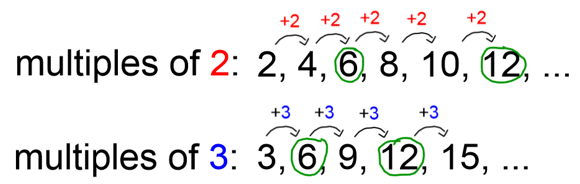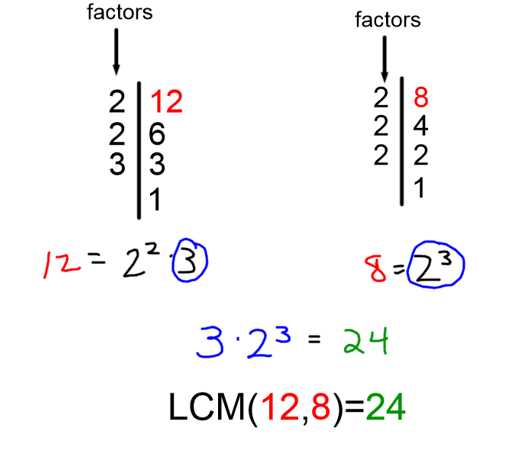Smartick is a fun way to learn math!Dec21

# How to Calculate Least Common Multiple

In this post, we are going to see what the least common multiple is and how to calculate it.

The least common multiple (LCM) of two or more numbers is the smallest number (not counting 0) which is a multiple of all of the numbers.

In order to better understand this definition, we are going to look at all of the terms:

• Multiple: the multiples of a number are the numbers you get when you add a number to itself repeatedly.• Common Multiple: A common multiple is a number than is a multiple of two or more numbers; in other words, it is a common multiple of all those numbers.

Following with the previous example, we are going to see the common multiples of 2 and 3.You will see that there are common multiples between two and three, which are emphasized in green in the picture: 6 and12.  Keep in mind that the multiples are infinite and we have only shown the first two in this example.

• Least Common Multiple: the least common multiple is the smallest of the common multiples.

Following with the previous example, if the common multiples of 2 and three 3 were 6 and 12, the least common multiple is 6, since it is smaller than 12.

To continue, we are going to see how to calculate the least common multiple.  You can use two methods.

### First method to calculate the least common multiple:

Is what we used before, in other words, we write the first multiples of each number, we note the multiples that are common and we choose the least common multiple.

### Second method to calculate the least common multiple:

The first thing we have to do is break down the prime factors of each number.  After, we will have to choose the common factors and not the greatest common to the greatest exponent, and finally, we have to multiple the chosen factors.

We are going to look at an example of this, calculating the LCM of 12 and 8.We are going to break down 12 and 8 into prime factors:

12 = 22 x 3          8 = 23

Now, we take the highest power of each prime factor in the prime factorization

and multiply them: 23 x 3 = 8 x 3 = 24

So the least common multiple of 12 and 8 is 24.

You can practice some online exercises about LCM and more elementary math in Smartick. Try it for free!

Fun is our brain’s favorite way of learning
Diane Ackerman
Smartick is a fun way to learn math
• 15 fun minutes a day
• Millions of students since 2009• Jibreel Abdullahi MusaOct 13 2021, 10:56 AM

thanks

• Aliyu Isah sidiAug 10 2021, 3:24 PM

Like the Page

• Azeez olawunmiApr 20 2021, 11:44 AM

Fantastic

• ArjunMar 21 2021, 11:44 AM

Super

• ZULKALLAINI MUHAMMADFeb 09 2021, 5:04 PM

THANK YOU SO MUCH

• DeepikaDec 22 2020, 1:13 AM

L.c.m. 6th class 12 20 25 30

Very nice one.

• GuibiSep 05 2020, 11:19 AM

Useful really

• abba kaka lawanMay 12 2019, 6:01 PM

hi, guys i’m a new friend of yours and i’m here to learn mathematics.

• V.S.MalhotraAug 16 2018, 1:28 AM

what is the class level in a school at which students are expected to have the ability to solve problems involving use of LCM ?